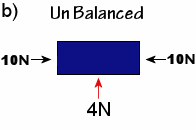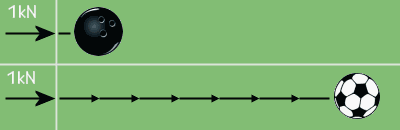## Newton's Laws Of Motion - Engineering Science

Inertia
Inertia can be described as the reluctance of a stationary object to change its state of motion or of a moving object to change its velocity or speed. Inertia is dependent on the mass of the object.
A force must be applied to an object to overcome its inertia; this could be friction, gravity or any other opposing force.

Newton’s 1st law of motion:
Every object in a state of uniform motion remains in that state of motion unless an external force is applied to it.

A change in the state of motion of an object is caused by a force. If forces are acting on a body and it remains stationary or continues to move at a constant velocity then the forces must be balanced, this means that the resultant forces must equal zero.  An object can only change its velocity when a resultant force acts upon it which causes the originally balanced resultant forces to become un balanced.

In the images below it’s clear that the forces are balanced in 'a)' and the blue rectangle would remain stationary. In 'b)' the blue rectangle would move in the direction of the red arrow this is because the unbalanced resulting force of 4N is acting in this direction.Newton’s 2nd law of motion:
When a resultant force acts on an object it will cause it to accelerate, the rate of acceleration is dependent on the applied force, the direction of acceleration is dependent on the direction of the applied force. The rate of acceleration is also dependent on the mass of the body on which the applied force is acting on. For example a force applied to a football would cause a much greater acceleration than if the same force were applied to a heavy weighted bowling ball.The relationship between an object's mass (m), its acceleration (a), and the applied force (F) is;
F = ma.
Or
a=F/m

The equation of motion defines the unit of force, the Newton (N), as the force required to produce an acceleration of 1 m s¯² in a body of mass 1kg.

Next Page >>>

EngineerStudent.co.uk © 2019